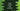# How to use pi value in Java with example## How to use pi value in Java:

Mathematical constant PI or π is already defined in the Math class or java.lang.Math class in Java. π is the ratio of the circumference of a circle to its diameter and it is a constant value.

It is a public static final double value and it is defined as like below:

``public static final double PI = 3.14159265358979323846;``

In this post, we will learn how to use this value in different ways.

### Use PI without importing Math module:

Since Math class is defined in the java.lang package, we can use its methods and other constants without importing this class. Also, since PI is a

The below program is printing the value of PI without using any imports:

``````public class Main{
public static void main(String[] args){
System.out.println(Math.PI);
}
}``````

If you run this program, it will print the value of PI.

### Use PI with static import:

Another way is to use static import. We can use static import to import the Math module and its constant can be accessed with using Math. For example:

``````import static java.lang.Math.*;

public class Main{
public static void main(String[] args){
System.out.println(PI);
}
}``````

Here,

• We are using static import, import static java.lang.Math.* and it imports all methods and constants defined in the Math class.
• We are accessing the value of PI without using Math.

### Use static import to import only PI:

The above example imports all methods and constants defined in the Math class. But, we can also import PI. We are not using any other methods or constants from the Math class. So, insted we can import only PI as like below:

``````import static java.lang.Math.PI;

public class Main{
public static void main(String[] args){
System.out.println(PI);
}
}``````

Instead of *, we are using PI to import it directly from the Math class. It also uses static import.

### Example of PI:

Let’s take an example of PI. PI is used to find the area of a circle. So, if we are writing a program in Java to calculate the area of a circle, we can use the Math module to read the PI value.

The formula to calculate the area of a circle is:

``Pi * R * R``

Let’s write a program that take the radius as input and prints the area.

``````import java.util.Scanner;

import static java.lang.Math.PI;

public class Main {
public static void main(String[] args) {
double r, area;
Scanner sc = new Scanner(System.in);

System.out.println("Enter the radius of the circle: ");
r = sc.nextFloat();

area = PI * r * r;

System.out.println("Area of the circle is: " + area);
}
}``````

It will give output as like below:

``````Enter the radius of the circle:
12
Area of the circle is: 452.3893421169302

Enter the radius of the circle:
10
Area of the circle is: 314.1592653589793``````

It is taking the radius as input from the user and printing the area. We are using static import to import PI and using its value, we are calculating the value of area.